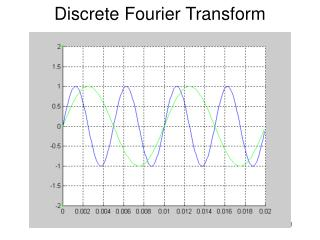DownloadDownload PresentationDiscrete Fourier Transform

# Discrete Fourier Transform

Download Presentation## Discrete Fourier Transform

- - - - - - - - - - - - - - - - - - - - - - - - - - - E N D - - - - - - - - - - - - - - - - - - - - - - - - - - -
##### Presentation Transcript

1. Discrete Fourier Transform

2. Multiply element-by-element

3. Cumulative sum shows:

4. 2 signals of same frequency and phase

5. Multiply element-by-element

6. Non-zero cumulative sum

7. Same frequency but /2 phase difference

8. Element-by element product with both sine and cosine waves

9. Cumulative sums

10. Wave: partly sine, partly cosine

11. Element-by-element multiplication

12. Cumulative sum

13. dftsimp2demo(f, fs, timelen, amp) dftsimp2demo(200, 1000, 0.02, 1)

14. dftsimp2demo(f, fs, timelen, amp) dftsimp2demo(200, 1000, 0.05, 1)

15. dftsimp2demo(f, fs, timelen, amp) dftsimp2demo(200, 10000, 0.05, 1)

16. dftcomplex2demo(f1, f2, fs, timelen, a1, a2) dftcomplex2demo(200, 400, 10000, 0.02, 5, 4)

17. dftcomplex2demo(f1, f2, fs, timelen, a1, a2) dftcomplex2demo(200, 400, 10000, 0.02, 5, 4)

18. dftspeech2demo(wavfile, timelen) dftspeech2demo('atest.wav', 0.04)

19. dftspeech2demo(wavfile, timelen) dftspeech2demo('atest.wav', 0.04)

20. Use dB scale and frequencies to Fs /2

21. dftspeech2demo(wavfile, timelen) dftspeech2demo(‘itest.wav', 0.04)

22. dftspeech2demo(wavfile, timelen) dftspeech2demo(‘itest.wav', 0.04)

23. DFT Procedure • Given the window (frame) length, decide the base frequency • Multiply by sine wave at each multiple of base frequency • Multiply by cosine wave at each multiple of base frequency • Calculate magnitude and phase spectra using

24. Complex Exponential • Given the window (frame) length, decide the base frequency • Multiply by sine wave at each multiple of base frequency • Multiply by cosine wave at each multiple of base frequency • Calculate magnitude and phase spectra using

25. Compact Formulae • DFT • IDFT